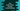## Java LinkedHashMap example : create and iterate through a LinkedHashMap :

LinkedHashMap is hash table and linked list implementation of the Map interface with predictable iteration order. It maintains a doubly linked list through all entries. The iteration order is normally the same order in which the keys are inserted into the map.

In this example, we will learn how to create and iterate through a LinkedHashMap in Java :

### Java program :

``````import java.util.*;

public class Main {

public static void main(String[] args) {
//1

//2
hashMap.put("one", 1);
hashMap.put("two", 2);
hashMap.put("three", 3);
hashMap.put("four", 4);
hashMap.put("five", 5);

//3
System.out.println("Printing elements of the LinkedHashMap : ");

//4
Set set = hashMap.entrySet();

//5
Iterator iterator = set.iterator();

//6
while (iterator.hasNext()) {
//7
Map.Entry item = (Map.Entry) iterator.next();

//8
System.out.println("Key = " + item.getKey() + " Value = " + item.getValue());
}

}

}``````

### Explanation :

The commented numbers in the above program denotes the step number below :

1. Create one LinkedHashMap with String keys and Integer values.
2. Insert five different values to the LinkedHashMap.
3. Print out the elements of the LinkedHashMap.
4. entrySet() method returns a Set view of the mapping contained in the LinkedHashMap. We will iterate through this Set. Assign this value to the variable set.
5. Create one Iterator to iterate through the set.
6. Run one while loop to iterate through the Set.
7. The return value of entrySet() is Set<Map.Entry<K,V>>. First, convert the next variable of the iterator to Map.Entry format and save it in the item variable.
8. Print out the key and value of the Set using getKey() and getValue() method. The output should be in the same order as the input lines.

### Output :

``````Printing elements of the LinkedHashMap :
Key = one Value = 1
Key = two Value = 2
Key = three Value = 3
Key = four Value = 4
Key = five Value = 5``````

source : oracle docs